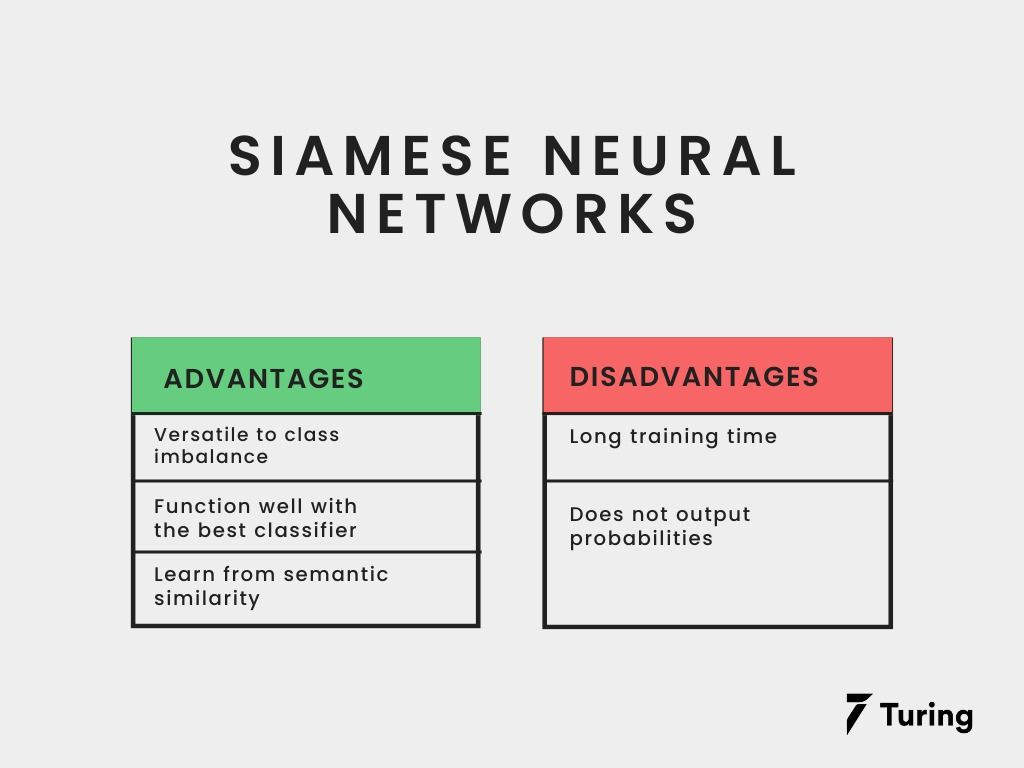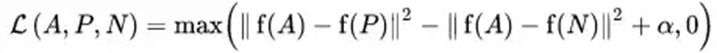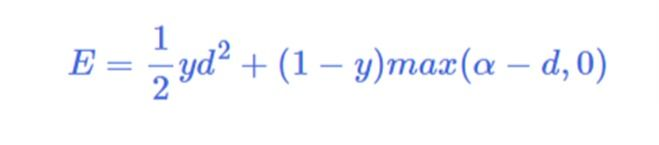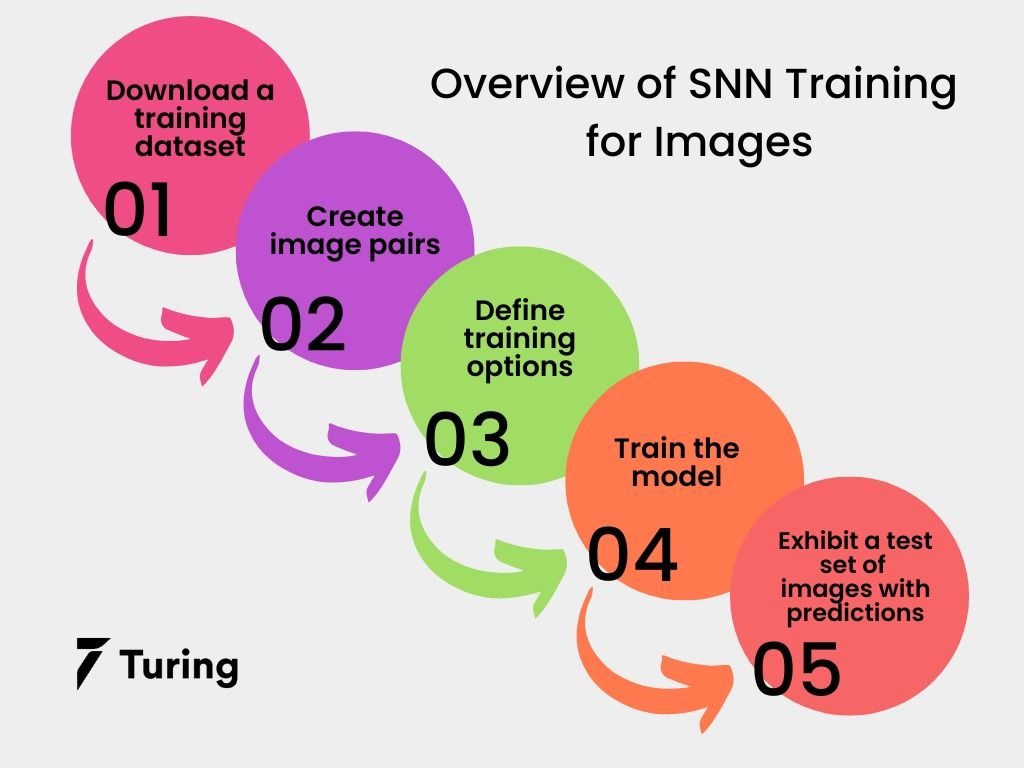# How Siamese Neural Networks Work With Image ProcessingNeural networks can be used for virtually any task, provided there is data. But for certain others, such as image processing for signature verification or facial recognition, we cannot rely on obtaining and processing more data. To solve these issues, we need to have a novel form of neural network architecture known as Siamese neural networks (SNNs). This article will examine how SNNs work and how they can be trained for image processing.

## What is a Siamese network?

A Siamese network is capable of using just a few images to get near-precise predictions. The capacity to learn from minute data has made it popular in the field of data science.

A Siamese neural network is a category of neural network architecture that features two or more undistinguishable sub-networks. This means that they contain an identical configuration of weights and parameters.

Parameter updating is reflected across subnetworks and is usually used to detect relationships between inputs by contrasting their feature vectors.

Normally, a neural network is built to learn how to predict multiple classes. But this can be problematic when we want to remove or add new classes to our data. The only way out is to update the network and retrain it again on the entire dataset. To make matters even more difficult, we need a huge dataset to train for deep neural networks. However, SNN learns a similarity function which enables it to ascertain if two images are identical. This process allows us to classify new data classes without the need to retrain the neural network.

## Pros and cons of Siamese neural networksThe following are the benefits and drawbacks of Siamese neural networks:

Pros

• Less prone to class imbalance

One-shot learning enables the subnetworks to be more efficient with fewer images for every class.

• Function well with the best classifier

SNNs’ learning mechanism is a little different from other classification models. But averaging it with a particular classifier is better than averaging two interrelated supervised models.

• Learn from semantic similarity

One of the biggest advantages of using SNN is its ability to learn from semantic similarity. This neural network learns the relative positioning of embeddings to group similar objects together.

Cons

For all their features and benefits, SNNs also have several downsides.

• Require a lot of training time

Perhaps the biggest drawback of Siamese neural networks is that they require more time to train compared to normal neural networks. This is because SNNs learn by comparing pairs of items/images which leads to more time consumption.

• Does not output probabilities

SNNs learn by pairwise comparison of the items/images to find similarities. Thus, the prediction too is based on this comparison and not on probabilities like other learning models.

## Supporting functions

Siamese neural networks cannot use the standard loss functions like other models since they learn by comparison and finding similarities. The two main types of loss functions used are triplet loss and contrastive loss.

### Triplet loss

This function involves comparing an anchor input to a positive input and negative input. The goal is to reduce the distance from the anchor input to the positive input, while the distance from the anchor input to the negative input is increased.This is the mathematical representation of triplet loss. Here the term α is used to “extend” the distance between the triplet pairs. f(A), f(P), and f(N) are feature embeddings of anchor, positive, and negative images.

When training SNNs, we feed this image triplet (anchor image, negative image, positive image) (anchor image, negative image, positive image) in the model, which fine-tunes the model to lower the distance between the anchor and positive images, while increasing the distances for negative images.

### Contrastive lossThis is one of the most popular and commonly used loss functions for Siamese neural networks. d is the euclidean distance and α is the margin. Contrastive loss functions are distance-based learning methods since the Euclidean distance between the image embeddings is used to calculate the loss.

## Training a Siamese neural network to work on imagesNext, we'll look at how to train an SNN with two similar subnetworks with the same unique structure, weights, and parameters. As mentioned, SNNs are generally utilized in operations involving finding similarity or dissimilarity between two items.

SNNs are often useful when there are multiple classes but only a few observations of each. As a result, there is inadequate data to train a conventional neural network to categorize these photos into classes. With SNNs, this lack of data is no issue when classifying images into their respective categories.

We will use the Omniglot dataset to train an SNN to compare a set of photos. The dataset contains 1623 handwritten characters for 50 alphabets, divided into a 30:20 ratio for training and testing. Each character is also written by 20 different people hired on Amazon’s Mechanical Turk. In this article, we will train our network to identify whether two characters are of the same alphabet or not.

After downloading the dataset, we use the function imageDatastore to load the images, and then manually set the image labels by parsing the file names.

``````Data = imageDatastore(dataTrain,LabelSource="none");

F = Data.Files;
parts = split(F,filesep);
L = join(parts(:,(end-2):(end-1)),"-");
Data.Labels = categorical(L);
``````

Our test set contains black and white handwritten letters from 30 alphabets and 20 observations for every letter. The images are sized 105x105x1 with pixel values ranging from 0 to 1.

### Step 2: Create image pairs

Divide the dataset into pairs of similar or dissimilar images. In this case, similar photos are merely different handwritten variations of the same character with the same label, whereas dissimilar images are separate characters with different labels.

We’ll use the getSiameseBatch method to generate random pairs of the same or different alphabets. These pairs are denoted by Pair1 and Pair2. If the pairs are of the same alphabet, pair_label is set to 1, else 0.

We will create a sample of five image pairs.

``````[Pair_1,Pair_2,pair_label] = getSiameseBatch(Data,10);
``````

Let's view the generated image pairs.

``````for i = 1:10
if pair_label(i) == 1
Label = "similar";
else
Label = "dissimilar";
end
subplot(2,5,i)
imshow([Pair_1(:,:,:,i) Pair_2(:,:,:,i)]);
title(Label)
end
``````

Siamese architecture

Two pictures must go via one of two comparable subnetworks with equal weights in order for the network to compare them. The subnetworks transform the 105x105x1 pictures into 4096-dimensional vectors throughout this procedure.

The 4096-dimensional representations of images will be the same for those in the same class. As a result, the subnetworks' output feature vectors are combined by subtractions before being processed with a fullyconnect function that only produces one output. The Siamese neural network prediction of whether the pair of pictures are similar or different is then shown using a sigmoid function which turns this output to odd values between 0 and 1.

The network is updated during the course of the training using the binary cross-entropy loss between the true label and the network prediction.

Here is the resulting code.

``````network_layers = [
imageInputLayer([105 105 1],Normalization="none")
convolution2dLayer(10,64,WeightsInitializer="narrow-normal",BiasInitializer="narrow-normal")
reluLayer
maxPooling2dLayer(2,Stride=2)
convolution2dLayer(7,128,WeightsInitializer="narrow-normal",BiasInitializer="narrow-normal")
reluLayer
maxPooling2dLayer(2,Stride=2)
convolution2dLayer(4,128,WeightsInitializer="narrow-normal",BiasInitializer="narrow-normal")
reluLayer
maxPooling2dLayer(2,Stride=2)
convolution2dLayer(5,256,WeightsInitializer="narrow-normal",BiasInitializer="narrow-normal")
reluLayer
fullyConnectedLayer(4096,WeightsInitializer="narrow-normal",BiasInitializer="narrow-normal")];

LG = layerGraph(network_layers);
``````

### Step 3: Define Siamese network loss function

We use the modelLoss function to output the loss values as well as the gradients of the loss. The function takes the fullyconnect function parameter structure, the Siamese subnetwork network, and a batch of input datasets X1 and X2 along with their labels operation, pair_labels.

SNN aims to identify the difference between input X1 and X2. The network output is a probability ranging from 0 to 1, with 1 being completely same, and 0 being no similarity.

### Step 4: Define training options

Define the options used for training. In this case, to train for 10000 iterations as shown below:

``````epochs = 10000;
size_batch = 180;
``````

Next, we define the ADAM optimization options, setting rate to 0.00006, the gradient decay factor to 0.9, and the squared gradient decay factor (decaySq) to 0.99 as illustrated below:

``````rate = 6e-5;
decay = 0.9;
decaySq = 0.99;
``````

### Step 5: Train the model

To initialize the training process, plot using the code below:

``````figure
col = colororder;
loss_chart = animatedline(Color=col(2,:));
ylim([0 inf])
xlabel("Iteration")
ylabel("Loss")
grid on
``````

Now to initialize the ADAM solver parameters:

``````TAsub = []
TAsqsub = []
TApara = []
TAsqPara = []
``````

To train the model, we use a traditional training loop to loop over the dataset while updating the network parameters after each iteration.

For each iteration,

• Mine a group of image labels and pairs using the function getSiameseBatch.
• Convert the training dataset to dlarray object to specify the dimension labels – channel, batch - ‘CB’ for labels and – spatial, spatial, channel, batch – ‘SSCB’ for the image data.
• Estimate the model gradients and loss using the functions modelLoss and dlfeval.
• Use the adamupdate operator to update the network.
``````start = tic;

# Looping
for loops = 1:epochs

[X1,X2,pair_label] = getSiameseBatch(Data,size_batch);

X1 = dlarray(X1,"SSCB");
X2 = dlarray(X2,"SSCB");

[Ls,g_sub,g_par] = dlfeval(@modelLoss,net,fcParams,X1,X2,pair_label);

TAsub,TAsqsub,loops,rate,decay,decaySq);

TApara,TAsqPara,loops,rate,decay,decaySq);

D = duration(0,0,toc(start),Format="hh:mm:ss");
Ls_1 = double(Ls);
drawnow
end
``````

### Step 6: Exhibit a test set of images with predictions

Next, we generate a sample set of picture pairings to experiment with to see if the SNN accurately predicted similar and different photos. To determine the prediction for each test pair, we utilize the function predictSiamese.

We output the picture pairings together with the predictions, probabilities, and a label designating whether or not the network correctly predicted them.

Here is an example:

``````#loading test data using imagedatastore
img_ds = imageDatastore(Test_data,IncludeSubfolders=true, LabelSource="none");

files = img_ds.Files;
parts = split(files,filesep);
labels = join(parts(:,(end-2):(end-1)),"_");
img_ds.Labels = categorical(labels);
test_set = 10;

[x1_test,x2_test,pair_labelTest] = getSiameseBatch(img_ds,test_set);
To convert the predictions to zero (0) or one (1),
Y_predicted = round(YScore);
To mine the data to plot,
x1_test = extractdata(x1_test);
x2_test = extractdata(x2_test);
To plot images along with the predicted score and label,
fig = figure;
tiledlayout(2,5);
fig.Position(3) = 2*fig.Position(3);

predicted_labels = categorical(Y_predicted,[0 1],["dissimilar" "similar"]);
target_labels = categorical(pair_labelTest,[0 1],["dissimilar","similar"]);

for i = 1:numel(pair_labelTest)
nexttile
imshow([x1_test(:,:,:,i) x2_test(:,:,:,i)]);

title(
print("Target {} \n".format(target_labels(i)))
print("Predicted {} \n".format(predicted_labels(i)))
print("Score {} \n".format(YScore(i)))
)
end
``````

The Siamese network will compare the images to predict their similarity, despite the fact that all the images used in the test were not in the training data.

## Conclusion

Most of us have heard of classification and regression problems but there is a third sort of problem called similarity questions that require us to determine whether two items are similar or not. Similarity learning is a subfield of supervised machine learning in which the goal is to learn a similarity function that calculates and returns a similarity value based on how similar or related two items are. When the objects are similar, a greater similarity score is returned and when the objects are distinct, a lower similarity value is returned.

In this article, we applied similarity learning using SNNs to compare different scripts from the Omniglot dataset. There are numerous applications for this, ranging from facial recognition to signature comparison. Since the amount of data necessary to train such networks is also quite small, SNNs are a very viable paradigm with huge potential in the future.

## Author

•### Turing

Author is a seasoned writer with a reputation for crafting highly engaging, well-researched, and useful content that is widely read by many of today's skilled programmers and developers.

Siamese networks are made up of two identical neural networks, each of which takes one of the two input images. The last layers of the two networks are then sent into a contrastive loss function which computes the degree of similarity between the two images.

Siamese networks are used to apply similarity learning, i.e., to find where there’s a similarity of patterns and structures in two images.

A Siamese neural network, also known as a twin neural network, is a type of artificial neural network that employs the same weights to compute equivalent output vectors from two distinct input vectors simultaneously.### Press

What's up with Turing? Get the latest news about us here.### Blog